# Grassmann elements and geometric algebra Λ(V)

Definition (Vector space $\Lambda^1 V = V$ is a field's $\mathbb K$-module instance). Let $V$ be a $\mathbb K$-module (abelian group with respect to $+$) with an element $1\in\mathbb K$ such that $1V = V$ by scalar multiplication $\mathbb K\times V\rightarrow V$ over field $\mathbb K$ satisfying

1. $a(x+y) = ax+ ay$ distribution of vector addition,
2. $(a+b)x = ax + bd$ distribution of field addition,
3. $(ab)x = a(bx)$ associative compatibility.

In the software package Grassmann, an underlying generating vector space is also synonymous with the term <:TensorBundle (an abstract type).

Definition (Linear dependence). Let $V$ be a vector space over field $\mathbb K$, then the set $\{v_i\}_i$ is linearly dependent if and only if $\sum_{i=1}^n k_iv_i = 0$ for some $0\ne k\in\mathbb K^n$.

Definition ($\wedge$-product annihilation). For a linearly dependent set $\{v_i\}_1^n\subset V$

$$$v_1\wedge v_2\wedge\dots\wedge v_n = 0.$$$

Initially, it is enough to understand that $\wedge:\Lambda^n V\times\Lambda^m V\rightarrow\Lambda^{n+m}V$ is an operation which is zero for linearly dependent arguments. However, this idea comes from extending Grassmann's product $v_i\wedge v_j = -v_j\wedge v_i \implies v_i\wedge v_i = 0 = -v_i\wedge v_i$ to yield a tool for characterizing linear dependence.

Definition (Dimension $n$-SubManifold in $\Lambda^n V$). Hence, writing the product $v_1\wedge v_2\wedge\cdots\wedge v_n\ne0$ implies a linearly independent set $\{v_i\}_1^n\subseteq V$ isomorphic to an $n$-SubManifold.

With the product $\Lambda^0\Lambda^n V\times(v_1\wedge v_2\wedge\cdots\wedge v_n)\cong \mathbb K$ it is also clear that a 1-dimensional basis subspace is induced by any $n$-SubManifold.

Example. Therefore, $\mathbb K = \Lambda^0\mathbb K \cong \Lambda^1\mathbb K$ is a vector space or a 0-SubManifold.

Example. $\Lambda^n V$ is a vector space with $\Lambda^1\Lambda^n V = \Lambda^nV$ and $\Lambda^0\Lambda^nV = \Lambda^0V$.

Denote $V^* = V\backslash\{0\}$ as the set $V$ excluding the 0 element in next:

Definition (Direct sum $\oplus$). To consider a set of linearly independent spaces, let $\pi_i: V\rightarrow V_i$ be projections with vector space $V_i\subset V$, define

$$$V_1\oplus V_2\oplus\cdots\oplus V_n = V \iff \bigwedge : V_1^*\times V_2^*\times\cdots\times V_n^* \rightarrow \Lambda^n V^* .$$$

DirectSum of a full non-zero product implies an $n$-SubManifold.

Ddefinition Grade-$m$ projection is defined as $\{\Lambda V\,\}_m = \Lambda^m V$ such that

$$$\Lambda V = \bigoplus_{m=0}^n \langle\Lambda V\,\rangle_m = \Lambda^0V\oplus\Lambda^1V\oplus\cdots\oplus\Lambda^nV, \qquad \langle\Lambda V\,\rangle_m = \bigoplus_{m=1}^{n\choose m}\mathbb K.$$$

Note that $\dim \{\Lambda V\,\}_m = {n\choose m}$ and hence $\dim\Lambda V = \sum_{m=0}^n {n\choose m} = 2^n$.

Example (Combinatorics of $\mathcal P(V)$ and hypergraphs $\subseteq P(V)\backslash\{\emptyset\}$). Let $v_1,v_2,v_3 \in\mathbb R^3$, then the power set of elements is:

$$$\mathcal P(\mathbb R^3) = \{\emptyset,\{v_1\},\{v_2\},\{v_3\},\{v_1,v_2\},\{v_1,v_3\},\{v_2,v_3\},\{v_1,v_2,v_3\}\}$$$

Form a direct sum over the elements of $\mathcal P(V)$ with $\wedge$ to define $\Lambda V$, e.g.

$$$\Lambda(\mathbb R^3) = \Lambda^0(\mathbb R^3)\oplus\Lambda^1(\mathbb R^3)\oplus\Lambda^2(\mathbb R^3)\oplus\Lambda^3(\mathbb R^3)$$$$$$\overbrace{v_\emptyset}^{\Lambda^0\mathbb R}\oplus \overbrace{v_1\oplus v_2\oplus v_3}^{\Lambda^1(\mathbb R^3)}\oplus\overbrace{(v_1\wedge v_2)\oplus (v_1\wedge v_3) \oplus (v_2\wedge v_3)}^{\Lambda^2(\mathbb R^3)}\oplus\overbrace{(v_1\wedge v_2\wedge v_3)}^{\Lambda^3(\mathbb R^3)}$$$

The Grassmann SubManifold elements $v_k\in\Lambda^1V$ and $w^k\in\Lambda^1V'$ are linearly independent vector and covector elements of $V$, while the Leibniz Operator elements $\partial_k\in L^1V$ are partial tangent derivations and $\epsilon_k\in L^1V'$ are dependent functions of the tangent manifold. Let $V\in\text{Vect}_{\mathbb k}$ be a TensorBundle with dual space $V'$ and the basis elements $w_k:V\rightarrow\mathbb K$, then for all $x\in V,c\in\mathbb K$ it holds: $(w^i+w^j)(x) = w^i(x)+w^j(x)$ and $(cw^k)(x) = cw^k(x)$ hold. An element of a mixed-symmetry TensorAlgebra{V} is a multilinear mapping that is formally constructed by taking the tensor products of linear and multilinear maps, $(\bigotimes_k \omega_k)(v_1,\dots,v_{\sum_k p_k}) = \prod_k \omega_k(v_1,\dots,v_{p_k})$. Higher grade elements correspond to SubManifold subspaces, while higher order function elements become homogenous polynomials and Taylor series.

julia> Λ(ℝ^3)
DirectSum.Basis{⟨+++⟩,8}(v, v₁, v₂, v₃, v₁₂, v₁₃, v₂₃, v₁₂₃)

julia> Λ(tangent(ℝ^2))
DirectSum.Basis{T¹⟨++₁⟩,8}(v, v₁, v₂, ∂₁, v₁₂, ∂₁v₁, ∂₁v₂, ∂₁v₁₂)

julia> Λ(tangent((ℝ^0)',3,3))
DirectSum.Basis{T³⟨¹²³⟩',8}(w, ϵ₁, ϵ₂, ϵ₃, ϵ₁₂, ϵ₁₃, ϵ₂₃, ϵ₁₂₃)

Combining the linear basis generating elements with each other using the multilinear tensor product yields a graded (decomposable) tensor SubManifold $\langle v_{i_1}\otimes\cdots\otimes v_{i_k}\rangle_k : V'^k\rightarrow\mathbb K$, where rank is determined by the sum of basis index multiplicities in the tensor product decomposition. The Grassmann anti-symmetric exterior basis is denoted by $v_{i_1\dots i_g}\in\Lambda^gV$ having the dual elements $w^{i_1\cdots i_g}\in\Lambda^gV'$, while the Leibniz symmetric basis will be denoted by $\partial_{i_1}^{\mu_1}\dots\partial_{i_g}^{\mu_g}\in L^gV$ with corresponding $\epsilon_{i_1}^{\mu_1}\dots\epsilon_{i_g}^{\mu_g}\in L^gV'$ adjoint elements. Combined, this space produces the full Leibniz tangent algebra $T^\mu V=V\oplus (\bigoplus_{g=1}^\mu L^g V)$ and the Grassmann exterior algebra $\Lambda V = \bigoplus_{g=1}^n\Lambda^g V$ with $2^n$ elements. The mixed index algebra $\Lambda(T^\mu V) = (\bigoplus_{g=1}^n\Lambda^g V)\oplus(\bigoplus_{g=1}^\mu L^g V)$ is partitioned into both symmetric and anti-symmetric tensor equivalence classes. Any mixed tensor SubManifold pair $\omega,\eta$ satisfies either

$$$\underbrace{\omega\otimes\eta = -\eta\otimes\omega}_{\text{anti-symmetric}} \qquad \text{or} \qquad \underbrace{\omega\otimes\eta = \eta\otimes\omega}_{\text{symmetric}}.$$$

For the oriented sets of the Grassmann exterior algebra, the parity of $(-1)^\Pi$ is factored into transposition compositions when interchanging ordering of the tensor product argument permutations. The symmetrical algebra does not need to track this parity, but has higher multiplicities in its indices. Symmetric differential function algebra of Leibniz trivializes the orientation into a single class of index multi-sets, while Grassmann's exterior algebra is partitioned into two oriented equivalence classes by anti-symmetry. Full tensor algebra can be sub-partitioned into equivalence classes in multiple ways based on the element symmetry, grade, and metric signature composite properties. Both symmetry classes can be characterized by the same geometric product.

julia> indices(Λ(3).v12)
2-element Vector{Int64}:
1
2

A higher-order composite tensor element is an oriented-multi-set $X$ such that $v_X = \bigotimes_k v_{i_k}^{\otimes\mu_k}$ with the indices $X = \left((i_1,\mu_1),\dots,(i_g,\mu_g)\right)$ and $|X|=\sum_k\mu_k$ is tensor rank. Anti-symmetric indices $\Lambda X\subseteq\Lambda V$ have two orientations and higher multiplicities of them result in zero values, so the only interesting multiplicity is $\mu_k\equiv1$. The Leibniz-Taylor algebra is a quotient polynomial ring $LV\cong R[x_1,\dots,x_n]/\{\prod_{k=1}^{\mu+1} x_{p_k}\}$ so that $\partial_k^{\mu+1}$ is zero. Typically the $k$ in a product $\left(\partial_{p_1}\otimes\cdots\otimes\partial_{p_k}\right)^{(k)}$ is referred to as the order of the element if it is fully symmetric, which is overall tracked separately from the grade such that $\partial_k\langle v_j\rangle_r = \langle\partial_kv_j\rangle_r$ and $(\partial_k)^{(r)}\omega_j = (\partial_kv_j)^{(r)}$. There is a partitioning into even grade components $\omega_+$ and odd grade components $\omega_-$ such that $\omega_++\omega_-=\omega$.

Grassmann's exterior algebra doesn't invoke the properties of multi-sets, as it is related to the algebra of oriented sets; while the Leibniz symmetric algebra is that of unoriented multi-sets. Combined, the mixed-symmetry algebra yield a multi-linear propositional lattice. The formal sum of equal grade elements is an oriented Chain and with mixed grade it is a MultiVector simplicial complex. Thus, various standard operations on the oriented multi-sets are possible including $\cup,\cap,\oplus$ and the index operation $\ominus$, which is symmetric difference operation.

By virtue of Julia's multiple dispatch on the field type $\mathbb K$, methods can specialize on the dimension $n$ and grade $G$ with a TensorBundle{n} via the TensorAlgebra{V} subtypes, such as SubManifold{V,G}, Simplex{V,G,B,𝕂}, Chain{V,G,𝕂}, SparseChain{V,G,𝕂}, MultiVector{V,𝕂}, and MultiGrade{V,G} types.

The elements of the Basis can be generated in many ways using the SubManifold elements created by the @basis macro,

julia> using Grassmann; @basis ℝ'⊕ℝ^3 # equivalent to basis"-+++"
(⟨-+++⟩, v, v₁, v₂, v₃, v₄, v₁₂, v₁₃, v₁₄, v₂₃, v₂₄, v₃₄, v₁₂₃, v₁₂₄, v₁₃₄, v₂₃₄, v₁₂₃₄)

As a result of this macro, all of the SubManifold{V,G} elements generated by that TensorBundle become available in the local workspace with the specified naming. The first argument provides signature specifications, the second argument is the variable name for the TensorBundle, and the third and fourth argument are prefixes of the SubManifold vector names (and covector basis names). By default, $V$ is assigned the TensorBundle and $v$ is the prefix for the SubManifold elements.

julia> V # Minkowski spacetime
⟨-+++⟩

julia> typeof(V) # dispatch by vector space
SubManifold{⟨-+++⟩, 4, 0x000000000000000f}

julia> typeof(v13) # extensive type info
SubManifold{⟨-+++⟩, 2, 0x0000000000000005}

julia> 2v1 + v3 # vector Chain{V,1} element
2v₁ + 0v₂ + 1v₃ + 0v₄

julia> 5 + v2 + v234 # MultiVector{V} element
5 + 1v₂ + 1v₂₃₄

It is entirely possible to assign multiple different bases with different signatures without any problems. In the following command, the @basis macro arguments are used to assign the vector space name to $S$ instead of $V$ and basis elements to $b$ instead of $v$, so that their local names do not interfere:

julia> @basis "++++" S b;

julia> let k = (b1 + b2) - b3
for j ∈ 1:9
k = k * (b234 + b134)
println(k)
end end
0 + 1v₁₄ + 1v₂₄ + 2v₃₄
0 - 2v₁ - 2v₂ + 2v₃
0 - 2v₁₄ - 2v₂₄ - 4v₃₄
0 + 4v₁ + 4v₂ - 4v₃
0 + 4v₁₄ + 4v₂₄ + 8v₃₄
0 - 8v₁ - 8v₂ + 8v₃
0 - 8v₁₄ - 8v₂₄ - 16v₃₄
0 + 16v₁ + 16v₂ - 16v₃
0 + 16v₁₄ + 16v₂₄ + 32v₃₄

Alternatively, if you do not wish to assign these variables to your local workspace, the versatile constructors of DirectSum.Basis{V} can be used to contain them, which is exported to the user as the method Λ(V),

julia> G3 = Λ(3) # equivalent to Λ(V"+++"), Λ(ℝ^3), Λ.V3
DirectSum.Basis{⟨×××⟩,8}(v, v₁, v₂, v₃, v₁₂, v₁₃, v₂₃, v₁₂₃)

julia> G3.v13 ⊖ G3.v12
1v₂₃

The multiplication product used: $*$ or $\ominus$ is the geometric algebraic product.

Definition. The geometric algebraic product is the oriented symmetric difference operator $\ominus$ (weighted by the bilinear form $g$) and multi-set sum $\oplus$ applied to multilinear tensor products $\otimes$ in a single operation.

$$$\omega_X\ominus \eta_Y = \underbrace{\overbrace{(-1)^{\Pi(X,Y)}}^{\text{orient parity}}\overbrace{\det\left[g_{\Lambda(X\cap Y)}\right]}^{\text{intersect metric}} (\overbrace{\bigotimes_{k\in \Lambda(X\ominus Y)} v^{i_k}}^{(X\cup Y)\backslash(X\cap Y)}}_{\Lambda^1-anti-symmetric,\, \Lambda^g-mixed-symmetry})\otimes (\underbrace{\overbrace{\bigotimes_{k\in L(X\oplus Y)} \partial_{i_k}^{\otimes\mu_k}}^{\text{multi-set sum}}}_{L^g-symmetric})$$$

Remark: The product symbol $\ominus$ will be used to denote explicitly usage of the geometric algebraic product, although the standard number product $*$ notation could also be used. The $\ominus$ choice helps emphasize that the geometric algebraic product is characterized by symmetric differencing of anti-symmetric indices.

julia> (1 + 2v34) ⊖ (3 + 4v34), (1 + 2v34) * (3 + 4v34), (1 + 2im) * (3 + 4im)
(-5 + 10v₃₄, -5 + 10v₃₄, -5 + 10im)

Symmetry properties of the tensor algebra can be characterized in terms of the geometric product by two averaging operations, which are the symmetrization $\odot$ and anti-symmetrization $\boxtimes$ operators. These products satisfy various MultiVector properties, including the associative and distributive laws.

Definition (Exterior product): Let $w_k\in\Lambda^{p_k}V$, then for all $\sigma\in S_{\sum p_k}$ define an equivalence relation $\sim$ such that

$$$\bigwedge_k \omega_k(v_{1},\dots,v_{p_k}) \sim (-1)^{\Pi(\sigma)}(\bigotimes_k \omega_k)(v_{\sigma(1)},\dots,v_{\sigma(\sum p_k)})$$$

if and only if $\ominus_k\omega_k = \boxtimes_k\omega_k$ holds. It has become typical to use the $\wedge$ product symbol to denote products of such elements as $\bigwedge\Lambda V \equiv \bigotimes\Lambda V/\sim$ modulo anti-symmetrization.

julia> v3 ∧ v4, v4 ∧ v3, v3 ∧ v3
(v₃₄, -1v₃₄, 0v)

Remark. Observe that the anti-symmetric property implies that $\omega\otimes\omega=0$, while the symmetric property neither implies nor denies such a property. Grassmann remarked in 1862 that the symmetric algebra of functions is by far more complicated than his anti-symmetric exterior algebra. The first part of the book focused on anti-symmetric exterior algebra, while the more complex symmetric function algebra of Leibniz was subject of the second multivariable part of the book. Elements $\omega_k$ in the space $\Lambda V$ of anti-symmetric algebra are often studied as unit quantum state vectors in a unitary probability space, where $\sum_k\omega_k\neq\bigotimes_k\omega_k$ is entanglement.

Definition (Reverse, involute, conjugate). The reverse of $\langle\omega\rangle_r$ is defined as $\langle\tilde\omega\rangle_r = (-1)^{(r-1)r/2}\langle\omega\rangle_r$, while the involute is $\langle\omega\rangle_r^\times=(-1)^r\langle\omega\rangle_r$ and clifford $\langle\omega\rangle_r^\ddagger$ is the composition of involute and reverse.

julia> clifford(v234) == involute(~v234)
true

Definition (Reversed product). Define the index reversed product $\ast$ which yields a Hilbert space structure:

$$$\omega\ast\eta = \tilde\omega\ominus\eta, \quad \omega\ast'\eta = \omega\ominus\tilde\eta, \qquad |\omega|^2 = \omega\ast\omega, \quad |\omega| = \sqrt{\omega\ast\omega}, \quad ||\omega|| = \text{Euclidean }|\omega|.$$$

Remark. Observe that $\ast$ and $\ast'$ could both be exchanged in abs, abs2, and norm; however, these are different products. The scalar product $\circledast$ is the scalar part, so $\eta\circledast\omega = \langle\eta\ast\omega\rangle$.

julia> 2v34 ⊖ 2v34, 2v34 * 2v34, 2v34 ∗ 2v34, 2v34 ⊛ 2v34 # (gp, gp, rp, sp)
(-4v, -4v, 4v, 4v)

julia> abs2(2v34), abs(2v34), norm(2v34) # application of reverse product
(4v, 2.0v, 2.0)

Definition (Inverse). $\omega^{-1} = \omega\ast(\omega\ast\omega)^{-1} = \tilde\omega/|\omega|^2$, with $\eta/\omega = \eta\ominus\omega^{-1}$ and $\eta\backslash\omega = \eta^{-1}\ominus\omega$.

julia> 1/v34, inv(v34) == ~v34/abs2(v34)
(-1.0v₃₄, true)

Definition (Sandwich product). This product can be defined as $\eta\oslash\omega = \omega\backslash\eta\ominus\omega^\times$. Alternatively, the reversed definition is $\eta^\times\ominus\omega/\eta$ or in Julia η>>>ω, which is often found in literature.

julia> (2v3+5v4) ⊘ v3 == inv(v3)*(2v3+5v4)*involute(v3)
true

Remark. Observe that it is overall more simple and consistent to use $\{\ast,\oslash\}$ operations instead of the reversed.

The real part $\Re\omega = (\omega+\tilde\omega)/2$ is defined by $|\Re\omega|^2 = (\Re\omega)^{\ominus2}$ and the imag part $\Im\omega = (\omega-\tilde\omega)/2$ by $|\Im\omega|^2 = -(\Im\omega)^{\ominus2}$, such that $\omega = \Re\omega+\Im\omega$ has real and imaginary partitioned by

$$$\langle\tilde\omega\rangle_r/\left|\langle\omega\rangle_r\right| = \sqrt{\langle\tilde\omega\rangle_r^2/\big|\langle\omega\rangle_r\big|^2} = \sqrt{\langle\omega\rangle_r\ast\langle\omega\rangle_r^{-1}} = \sqrt{\langle\tilde\omega\rangle_r/\langle\omega\rangle_r}=\sqrt{(-1)^{(r-1)r/2}} \in\{1,\sqrt{-1}\},$$$

which is a unique partitioning completely independent of the metric space and manifold of the algebra.

$$$\omega\ast\omega = |\omega|^2 = |\Re\omega+\Im\omega|^2 = |\Re\omega|^2+|\Im\omega|^2 + 2\Re(\Re\omega\ast\Im\omega)$$$

The radial and angular components in a multivector exponential are partitioned by the parity of their metric.

It is possible to assign the quaternion generators $i,j,k$ with

julia> i,j,k = hyperplanes(ℝ^3)
3-element Vector{Simplex{⟨+++⟩, 2, B, Int64} where B}:
-1v₂₃
1v₁₃
-1v₁₂

julia> i^2, j^2, k^2, i*j*k
(-1v, -1v, -1v, -1v)

julia> -(j+k) * (j+k)
2v⃖

julia> -(j+k) * i
0 - 1v₁₂ - 1v₁₃

Alternatively, another representation of the quaternions is

julia> basis"--"
(⟨--⟩, v, v₁, v₂, v₁₂)

julia> v1^2, v2^2, v12^2, v1*v2*v12
(-1v, -1v, -1v, -1v)

The parametric type formalism in Grassmann is highly expressive to enable the pre-allocation of geometric algebra computations for specific sparse-subalgebras, including the representation of rotational groups, Lie bivector algebras, and affine projective geometry. All of this is enabled by the psuedoscalar complement duality.

Definition (Poincare-Hodge complement $\star$): Let $\omega = w_{i_1}\wedge\dots\wedge w_{i_p}$ and $\star\omega = \widetilde\omega I$, then $\star : \Lambda^pV\rightarrow\Lambda^{n-p}V$.

Remark. While $\star\omega$ is complementrighthodge of $\omega$, the complementlefthodge would be $I\widetilde\omega$. The $\star$ symbol was added to the Julia language as unary operator for ease of use with Grassmann on Julia's v1.2 release.

With LightGraphs.jl, GraphPlot.jl, Cairo.jl, Compose.jl it is possible to convert Grassmann numbers into graphs.

using Grassmann, Compose # environment: LightGraphs, GraphPlot
x = Λ(ℝ^7).v123
Grassmann.graph(x+!x)
draw(PDF("simplex.pdf",16cm,16cm),x+!x)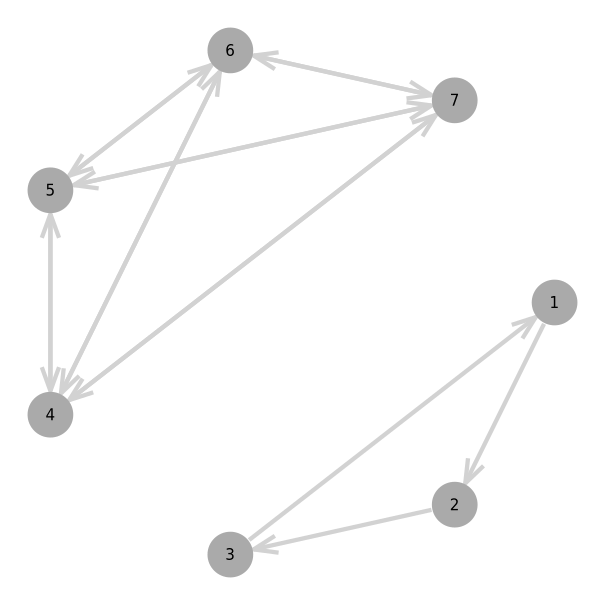Figure. Triangle with its tetrahedron complement $v_{123} + \star v_{123}$ in $\mathbb R^7$.

John Browne has discussed Grassmann duality principle in book, stating that every theorem (involving either of the exterior and regressive products) can be translated into its dual theorem by replacing the $\wedge$ and $\vee$ operations and applying Poincare duality (homology). Applying this Grassmann duality principle to the $\wedge$ product, let $P=\sum_kp_k$, $\{\omega_k\}_k\in\Lambda^{p_k}V$, then it is possible to obtain the co-product $\bigvee :\Lambda^{p_1}V_1\times\dots\times\Lambda^{p_g}V_g \rightarrow \Lambda^{P-(g-1)\#V}\bigcup_k V_k$. Grassmann's original notation implicitly combined $\wedge,\vee,\star$. The join $\wedge$ product is analogous to union $\cup$, the meet $\vee$ product is analogous to intersection $\cap$, and the orthogonal complement $\star\mapsto^\perp$ is negation. Together, $(\wedge,\vee,\star)$ yield an orthocomplementary propositional lattice (quantum logic):

$$$(\star\bigvee_k \omega_k)(v_1,\dots,v_P) = (\bigwedge_k\star\omega_k)(v_1,\dots,v_P) \quad DeMorgan's\,Law,$$$

where DeMorgan's law is used to derive tensor contractions.

However, this is only completely true for Euclidean algebras. In general, the original Grassmann (OG) complement must be used in DeMorgan's Law, while tensor contractions utilize the Hodge complement's metric.

Definition (Original Grassmann complement $|$). This operation is the same as $\star$ but is always Euclidean ($g\equiv 1$). In Julia it is also the ! method.

Interior contractions $\eta\cdot\omega = \eta\vee\star\omega$ need both $\star$ and $|$ complements. Of fundamental importance is the complement of a complement axiom:

Theorem. Let $\omega\in\Lambda^m V$, then $\star\star\omega = (-1)^{m(n-m)}\omega |I|^2$.

Foundationally important formulas include the Grassmann complement axiom with a Euclidean manifold: Corollary (Euclidean complement of a complement axiom). Let $\omega\in\Lambda^m(\mathbb R^n)$, then $\star\star\omega = (-1)^{m(n-m)}\omega$ since $|I|^2=1$.

The following lemma and corollary are helpful:

Lemma. Let $\omega\in\Lambda^mV$, then $I\vee\omega = \omega$.

Corollary. Obviously, $\tilde\omega I = I\cdot\omega$ since $I\cdot\omega = I\vee\star\omega = \star\omega = \tilde\omega I$.

Interior and exterior product with Hodge element

Theorem. Let $\omega\in\Lambda^m V$, then $(\omega\vee\star\omega)I = \omega\wedge\star\omega$.

Theorem. $\eta\wedge\star\omega = (\widetilde\omega\vee\star\widetilde\eta)I = (\widetilde\omega\cdot\widetilde\eta)I \iff \eta\cdot\omega = \eta\vee\star\omega = (\widetilde\omega\wedge\star\widetilde\eta)/I$.

Theorem. Let $\eta,\omega\in\Lambda^mV$, then $\tilde\eta\cdot\tilde\omega = \eta\cdot\omega$.

Corollary (Absolute value $|\omega|^2=\omega\cdot\omega$).

$$$(\omega\cdot\omega)I = \tilde\omega\wedge\star\tilde\omega = \tilde\omega\star\tilde\omega = \tilde\omega\omega I = |\omega|^2I \iff \omega\cdot\omega = \tilde\omega\omega$$$

The expressions can also be reversed: $\omega\wedge\star\omega = \omega\star\omega = \omega\tilde\omega I = |\omega|^2I$. However, when $\eta\in\Lambda^rV$ and $\omega\in\Lambda^sV$ are of unequal grade, then there exist several possible variations of graded contraction operations. Of course, the most natural option for the interior contraction is Grassmann's right contraction also written $\eta |\omega = \eta\vee\star\omega$. However, many authors such as Dorst \cite{dorst-inner} prefer the Conventional contraction, which is one of the other variations.

Contractionleft($\eta,\omega$)right($\eta,\omega$)
Grassmann$\langle\omega\rangle_s\vee\star\langle\eta\rangle_r = \langle\tilde\eta\omega\rangle_{s-r}$$\langle\eta\rangle_r\vee\star\langle\omega\rangle_s = \langle\tilde\eta\omega\rangle_{r-s} Reversed\langle\tilde\omega\rangle_s\vee\star\langle\tilde\eta\rangle_r = \langle\eta\tilde\omega\rangle_{s-r}$$\langle\tilde\eta\rangle_r\vee\star\langle\tilde\omega\rangle_s = \langle\eta\tilde\omega\rangle_{r-s}$
Conventional$\langle\omega\rangle_s\vee\star\langle\tilde \eta\rangle_r = \langle\eta\omega\rangle_{s-r}$$\langle\tilde \eta\rangle_r\vee\star\langle\omega\rangle_s = \langle\eta\omega\rangle_{r-s} Unconventional\langle\tilde \omega\rangle_s\vee\star\langle\eta\rangle_r = \langle\tilde \eta\tilde \omega\rangle_{s-r}$$\langle\eta\rangle_r\vee\star\langle\tilde\omega\rangle_s = \langle\tilde \eta\tilde \omega\rangle_{r-s}$
julia> (v1 + v2) ⋅ (1.5v2 + v3)
1.5v

Definition. Symmetrically define skew left $\lrcorner$ and right $\llcorner$ contractions $\langle\omega\rangle_r\cdot\langle\eta\rangle_s = \begin{cases} \omega\llcorner\eta=\omega\vee\star\eta & r\geq s \\ \omega\lrcorner\eta=\eta\vee\star\omega & r\leq s \end{cases}$. Note for $\omega,\eta$ of equal grade, $\omega\circledast\eta = \omega\odot\eta = \omega\cdot\eta = \omega\llcorner\eta = \omega\lrcorner\eta$ are all symmetric. In Julia, $\lrcorner$ is $<$ and $\llcorner$ is $>$.

julia> (G3.v1 + G3.v2) ⋅ (1.5G3.v2 + G3.v3)
1.5v

Definition. Let $\nabla = \sum_k\partial_kv_k$ be a vector field and $\epsilon = \sum_k\epsilon_k(x)w_k \in \Omega^1V$ be unit sums of the mixed-symmetry basis. Elements of $\Omega^pV$ are known as differential $p$-forms and both $\nabla$ and $\epsilon$ are tensor fields dependent on $x\in W$. Another notation for a differential form is $dx_k = \epsilon_k(x)w_k$, such that $\epsilon_k = dx_k/w_k$ and $\partial_k\omega(x) = \omega'(x)$.

julia> tangent(ℝ^3)(∇)
0v₁₂ + 0v₁₃ + 1∂₁v₁ + 0v₂₃ + 1∂₁v₂ + 1∂₁v₃

julia> (ℝ^3)(∇)
1v₁ + 1v₂ + 1v₃

Remark. The space $W$ does not have to equal $V\in\text{Vect}_{\mathbb K}$ above, as $\Omega^pV$ could have coefficients from $\mathbb K = LW$.

Definition. Define differential $d:\Omega^p V\rightarrow\Omega^{p+1}V$ and co-differential $\delta:\Omega^pV\rightarrow\Omega^{p-1}V$ such that

$$$\star d\omega = \star(\nabla\wedge\omega) = \nabla\times\omega, \qquad \omega\cdot\nabla = \omega\vee\star\nabla = \partial\omega =-\delta\omega.$$$

Vorticity curl of vector-field: $\star d(dx_1+dx_2+dx_3) = (∂_2 -∂_3)dx_1 + (∂_3 -∂_1)dx_2 + (∂_1 -∂_2)dx_3$.

julia> @basis tangent(ℝ^3,2,3); ⋆d(v1+v2+v3)
0 - 1∂₂v₁ + 1∂₃v₁ + 1∂₁v₂ - 1∂₃v₂ - 1∂₁v₃ + 1∂₂v₃

Boundary of 3-simplex, faces of simplex (oriented): $\partial(v_{1234}) = -\partial_4v_{123}+\partial_3v_{124}-\partial_2v_{134}+\partial_1v_{234}$.

julia> ∂(Λ(tangent(ℝ^4,2,4)).v1234)
0.0 - 1∂₄v₁₂₃ + 1∂₃v₁₂₄ - 1∂₂v₁₃₄ + 1∂₁v₂₃₄

These two maps have the special properties $d\circ d=0$ and $\partial\circ\partial = 0$ for any form $\omega$ and vector field $\nabla$. In topology there is boundary operator $\partial$ defined by $\partial\epsilon = \epsilon\cdot\nabla = \sum_k\partial_k\epsilon_k$ and is commonly discussed in terms the limit $\epsilon(x)\cdot\nabla\omega(x) = \lim_{h\rightarrow0} \frac{\omega(x+h\epsilon)-\omega(x)}{h}$, which is the directional derivative.

Theorem (Integration by parts & Stokes). Let $\nabla \in\Omega_1 V$ be a Leibnizian vector field operator, then $d,-\partial$ are Hilbert adjoint Hodge-DeRahm operators with

$$$\int_M d\omega\wedge\star\eta +\int_M \omega\wedge\star\partial\eta = 0, \qquad \langle d\omega\ast\eta\rangle =\langle\omega\ast-\partial\eta\rangle.$$$

Proof. Recall, $\partial\omega = \omega\cdot\nabla = \star^{-1}(\star\omega\wedge\star^2\nabla) = (-1)^n(-1)^{nk}\star d\star\omega$. Then substitute this into the integral $\int_M \omega\wedge(-1)^{mk+m+1}\star\star d\star\eta = (-1)^{km+m+1}(-1)^{(m-k+1)(k-1)}\int_M\omega\wedge d\star\eta$, and apply the identity $(-1)^{km+m+1}(-1)^{(m-k+1)(k-1)}=(-1)^k$ and $(-1)^k\int_M\omega\wedge d\star\eta = \int_M d(\omega\wedge\star\eta) - (-1)^{k-1}\omega\wedge d\star\eta = \int_M d\omega\wedge\star\eta$. Stokes identity can be proved by relying on a variant of the common factor theorem by Browne.

Theorem (Clifford-Dirac-Laplacian) Dirac operator is $(\nabla^2)^\frac12\omega = \pm\nabla\ominus\omega = \pm\nabla\wedge\omega \pm \nabla\cdot\omega = \pm d\omega\pm\partial\omega$.

$$$\nabla^2\omega = \nabla\wedge(\omega\cdot\nabla) + (\nabla\wedge\omega)\cdot\nabla) = \mp(\mp\omega\ominus\nabla)\ominus\nabla).$$$

Elements $\omega\in\mathcal H^p M = \{\nabla\omega = 0\mid\omega\in \Omega^pM\}$ are harmonic forms if $\nabla\omega = 0$ and hence both closed $d\omega=0$ and coclosed $\delta\omega=0$. Hodge decomposition: $\Omega^pM=\mathcal H^pM\oplus\text{im}(d\Omega^{p-1}M)\oplus\text{im}(\partial\Omega^{p+1}M)$.

julia> ω = 4.5v12 + 7.4v13
4.5v₁₂ + 7.4v₁₃ + 0.0∂₁v₁ + 0.0∂₂v₁ + 0.0∂₃v₁ + 0.0v₂₃ + 0.0∂₁v₂ + 0.0∂₂v₂ + 0.0∂₃v₂ + 0.0∂₁v₃ + 0.0∂₂v₃ + 0.0∂₃v₃ + 0.0∂₁₂ + 0.0∂₁₃ + 0.0∂₂₃

julia> V(∇^2)*ω == V(∇)*V(∇)*ω == d(∂(ω)) + ∂(d(ω))
true

Let $\nabla\in\Lambda^1V$, then $\omega = (\nabla\backslash\nabla)\ominus\omega = \nabla\backslash(d\omega + \partial\omega)$ where $\nabla\parallel\partial\omega$ and $\nabla\perp d\omega$. Let's reflect across the hyperplane $\star\nabla$, then $\nabla\backslash (d\omega-\partial\omega) = \nabla\backslash(d\omega-\partial\omega)\ominus(\nabla\backslash\nabla) = -\nabla^2\backslash(d\omega+\partial\omega)\ominus\nabla = -\nabla\backslash\omega\ominus\nabla$. Hence, reflection by hyperplane $\star\nabla$ has isometry $\omega\oslash\nabla$ which is a versor outermorphism.

Theorem (Cartan-Dieudonne). Every isometry of $V\rightarrow V$ is the composite of at most $k$ reflections across non-singular hyperplanes. Hence there exist vectors $\nabla_j$ such that

$$$(((\omega\oslash\nabla_1)\oslash\nabla_2)\oslash\cdots)\oslash\nabla_k = \omega\oslash(\nabla_1\ominus\nabla_2\ominus\dots\ominus\nabla_k)$$$

for any isometry element of the orthogonal group $O(p,q)$. Note that elements under transformations of this group preserve inner product relations. The even grade operators make up the rotational group, where each bivector isometry is a composition of two reflections.

Consider the differential equation $\partial_i\epsilon_j = \epsilon_j\oslash\omega$ with the solution $\epsilon_j(x) = \epsilon_j(0)\oslash e^{x_i\omega}$ where $\theta =2 x_i$ is the parameter of the Lie group. Then for a normalized $\omega$,

$$$e^{\theta\omega} = \sum_k \frac{(\theta\omega)^{\ominus k}}{k!} = \begin{cases} \cosh\theta+\omega\sinh\theta, & \text{if } \omega^2 = 1, \\ \cos\theta + \omega\sin\theta, & \text{if } \omega^2=-1, \\ 1+\theta\omega, & \text{if } \omega^2=0. \end{cases}$$$

Note that $\nabla\oslash e^{\theta\omega/2} = \nabla \ominus e^{\theta\omega}$ is a double covering when using the complex numbers in the Euclidean plane.

Due to GeometryTypes.jl Point interoperability, plotting and visualizing with Makie.jl is easily possible. For example, the vectorfield method creates an anonymous Point function that applies a versor outermorphism:

using Grassmann, Makie
basis"2" # Euclidean
streamplot(vectorfield(exp(π*v12/2)),-1.5..1.5,-1.5..1.5)
streamplot(vectorfield(exp((π/2)*v12/2)),-1.5..1.5,-1.5..1.5)
streamplot(vectorfield(exp((π/4)*v12/2)),-1.5..1.5,-1.5..1.5)
streamplot(vectorfield(v1*exp((π/4)*v12/2)),-1.5..1.5,-1.5..1.5)
@basis S"+-" # Hyperbolic
streamplot(vectorfield(exp((π/8)*v12/2)),-1.5..1.5,-1.5..1.5)
streamplot(vectorfield(v1*exp((π/4)*v12/2)),-1.5..1.5,-1.5..1.5)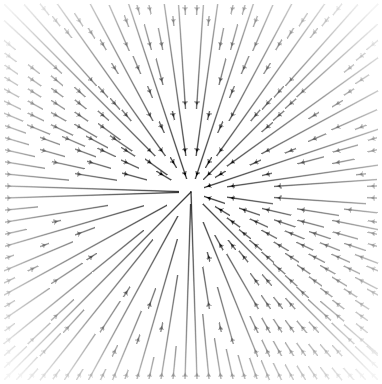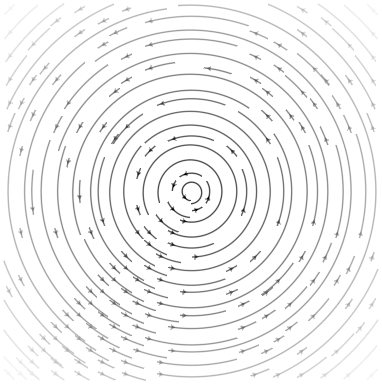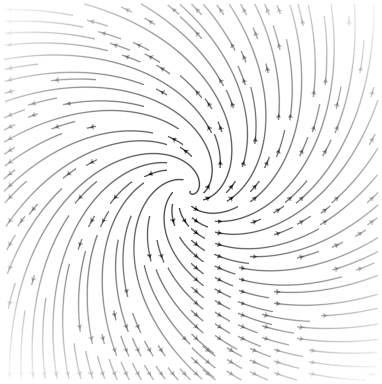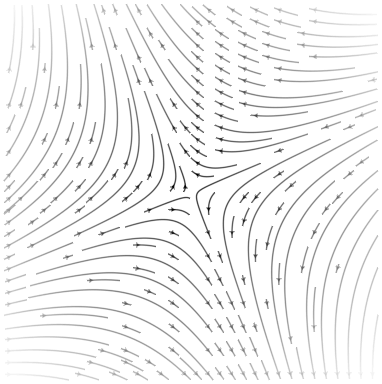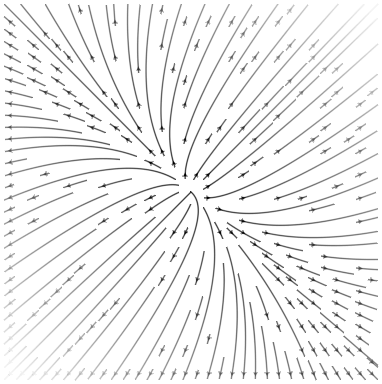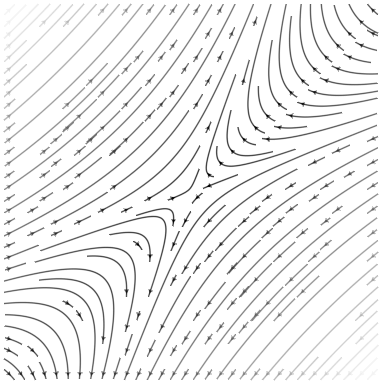using Grassmann, Makie
@basis S"∞+++"
f(t) = (↓(exp(π*t*((3/7)*v12+v∞3))>>>↑(v1+v2+v3)))
lines(V(2,3,4).(points(f)))
@basis S"∞∅+++"
f(t) = (↓(exp(π*t*((3/7)*v12+v∞3))>>>↑(v1+v2+v3)))
lines(V(3,4,5).(points(f)))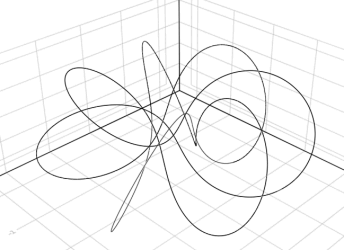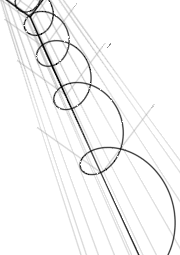using Grassmann, Makie; @basis S"∞+++"
streamplot(vectorfield(exp((π/4)*(v12+v∞3)),V(2,3,4)),-1.5..1.5,-1.5..1.5,-1.5..1.5,gridsize=(10,10))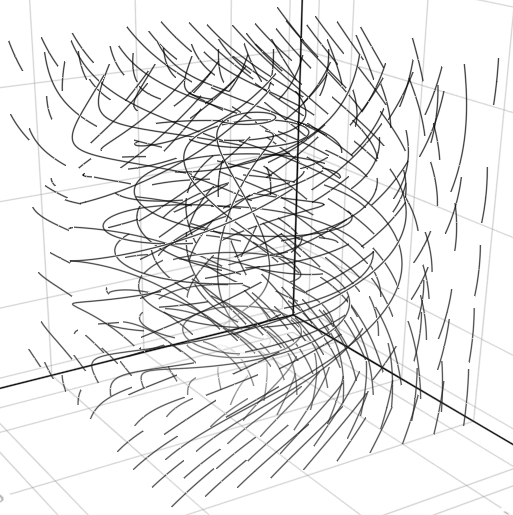using Grassmann, Makie; @basis S"∞+++"
streamplot(vectorfield(exp((π/4)*(v12+v∞3)),V(2,3,4),V(1,2,3)),-1.5..1.5,-1.5..1.5,-1.5..1.5,gridsize=(10,10))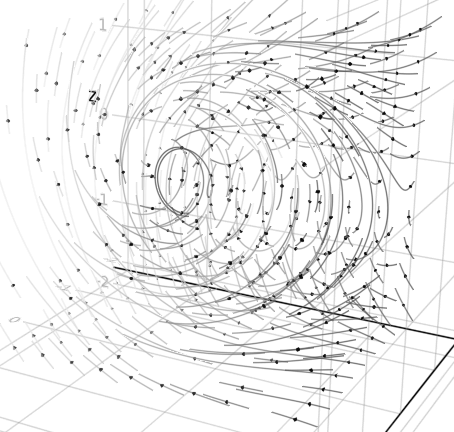using Grassmann, Makie; @basis S"∞+++"
f(t) = ↓(exp(t*v∞*(sin(3t)*3v1+cos(2t)*7v2-sin(5t)*4v3)/2)>>>↑(v1+v2-v3))
lines(V(2,3,4).(points(f)))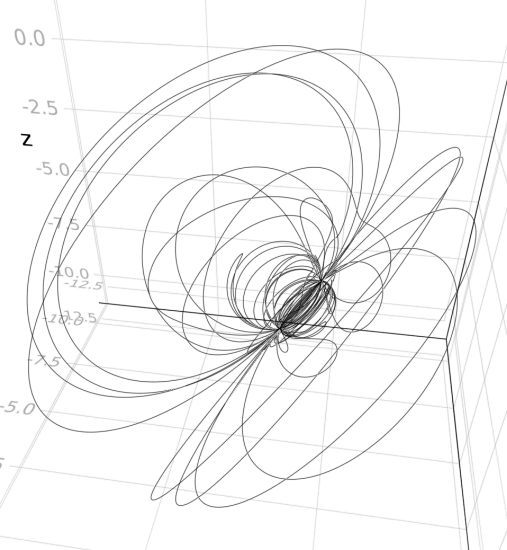using Grassmann, Makie; @basis S"∞+++"
f(t) = ↓(exp(t*(v12+0.07v∞*(sin(3t)*3v1+cos(2t)*7v2-sin(5t)*4v3)/2))>>>↑(v1+v2-v3))
lines(V(2,3,4).(points(f)))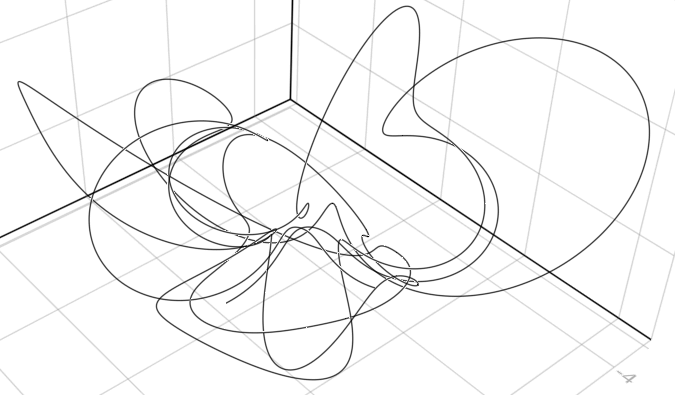As a result of Grassmann's exterior & interior products, the Hodge-DeRahm chain complex from cohomology theory has dimensional equivalence brought by the Grassmann-Poincare-Hodge complement duality,

$$$\mathcal H^{n-p}M \cong \frac{\text{ker}(d\Omega^{n-p}M)}{\text{im}(d\Omega^{n-p+1}M)}, \qquad \dim\mathcal H^pM = \dim\frac{\text{ker}(\partial\Omega^pM)}{\text{im}(\partial\Omega^{p+1}M)}.$$$

The rank of the grade $p$ boundary incidence operator is

$$$\text{rank}\langle\partial\langle M\rangle_{p+1}\rangle_p = \min\{\dim\langle\partial\langle M\rangle_{p+1}\rangle_p,\dim\langle M\rangle_{p+1}\}.$$$

Invariant topological information can be computed using the rank of homology groups, where $b_p(M)=\dim\mathcal H^pM$

$$$b_p(M) = \dim\langle M\rangle_{p+1} - \text{rank}\langle\partial\langle M\rangle_{p+1}\rangle_p - \text{rank}\langle\partial\langle M\rangle_{p+2}\rangle_{p+1}$$$

are the Betti numbers with Euler characteristic $\chi(M) = \sum_p (-1)^pb_p$.

Let's obtain the full skeleton of a simplical complex $\Delta(\omega)=\mathcal P(\omega)\backslash\Lambda^0(V)$ from the power set $\mathcal P(\omega)$ of all vertices with each subcomplex $\Delta(\partial(\omega))$ contained in the edge graph:

$$$\Delta(\omega) = \sum_{g=1}^n\sum_{k=1}^{n\choose g}\left(\text{abs}\langle\omega\rangle_{g,k} + \Delta\left(\text{abs}\,\partial\langle\omega\rangle_{g,k}\right)\right).$$$

Compute the value $\chi(\Delta(\omega))=1$ and $\chi(\Delta(\partial(\omega))) = \, ?$ for any Simplex $\omega$. As an exercise, also compute the corresponding betti numbers..

julia> [(χ(Δ(ω)),χ(Δ(∂(ω)))) for ω ∈ (Λ(ℝ5).v12,Λ(ℝ5).v123,Λ(ℝ5).v1234,Λ(ℝ5).v12345)]
4-element Vector{Tuple{Int64, Int64}}:
(1, 2)
(1, 0)
(1, 2)
(1, 0)

These methods can be applied to any MultiVector simplicial complex.

## Null-basis of the projective split

Let $v_\pm^2 = \pm1$ be a basis with $v_\infty = v_++v_-$ and $v_\emptyset = (v_--v_+)/2$ An embedding space $\mathbb R^{p+1,q+1}$ carrying the action from the group $O(p+1,q+1)$ then has $v_\infty^2 =0$, $v_\emptyset^2 =0$, $v_\infty \cdot v_\emptyset = -1$, and $v_{\infty\emptyset}^2 = 1$ with Minkowski plane $v_{\infty\emptyset}$ having the Hestenes-Dirac-Clifford product properties,

julia> using Grassmann; @basis S"∞∅++"
(⟨∞∅++⟩, v, v∞, v∅, v₁, v₂, v∞∅, v∞₁, v∞₂, v∅₁, v∅₂, v₁₂, v∞∅₁, v∞∅₂, v∞₁₂, v∅₁₂, v∞∅₁₂)

julia> v∞^2, v∅^2, v1^2, v2^2
(0v, 0v, 1v, 1v)

julia> v∞ ⋅ v∅, v∞∅^2
(-1v, 1v)

julia> v∞∅ * v∞, v∞∅ * v∅
(-1v∞, 1v∅)

julia> v∞ * v∅, v∅ * v∞
(-1 + 1v∞∅, -1 - 1v∞∅)

For the null-basis, complement operations are different:

$$$\star v_\infty = \star(v_++v_-) = (v_- + v_+)v_{1...n} = v_{\infty1...n}$$$$$$\star 2v_\emptyset = \star(v_--v_+) = (v_+ - v_-)v_{1...n} = -2v_{\emptyset1...n}$$$

The Hodge complement satisfies $\langle\omega\ast\omega\rangle I=\omega\wedge\star\omega$. This property is naturally a result of using the geometric product in the definition. An additional metric independent version of the complement operation is available with the ! operator,

$$$!v_\infty = !(v_++v_-) = (v_- - v_+)v_{1...n} = 2v_{\emptyset1...n}$$$$$$!2v_\emptyset = !(v_--v_+) = (v_+ + v_-)v_{1...n} = -v_{\infty1...n}$$$

For that variation of complement, $||\omega||^2 I = \omega\,\wedge\,!\omega$ holds.

julia> ⋆v∞, !v∞, ⋆v∅, !v∅
(1v∞₁₂, 2v∅₁₂, -1v∅₁₂, -0.5v∞₁₂)

julia> !v∞ * v12 == -2v∅, !v∅ * v12 == v∞/2
(true, true)

julia> ⋆v∞ * v12 == -v∞, ⋆v∅ * v12 == v∅
(true, true)

julia> v∞ * !v∞, v∅ * !v∅
(0 - 2v₁₂ + 2v∞∅₁₂, -0.0 + 0.5v₁₂ + 0.5v∞∅₁₂)

In this example, the null-basis properties from the projective split are shown.

julia> tangent(S"∞∅++",2,4)(∇^2)
0 + 1∂₃∂₃ + 1∂₄∂₄ - 2∂₁₂

## Differential forms and tangent algebra

Definition (Symmetric Leibniz differentials): Let $\partial_k = \frac\partial{\partial x_k}\in L_gV\,$ be Leibnizian symmetric tensors, then there is an equivalence relation $\asymp$ which holds for each $\sigma\in S_p$

$$$(\partial_p \circ \dots\circ \partial_1)\omega \asymp(\bigotimes_k \partial_{\sigma(k)})\omega \iff \ominus_k\partial_k = \bigodot_k\partial_k,$$$

along with each derivation $\partial_k(\omega\eta) = \partial_k(\omega)\eta + \omega\partial_k(\eta)$.

The product rule is encoded into Grassmann algebra when a tangent bundle is used, demonstrated here symbolically with Reduce by using the dual number definition:

julia> using Grassmann, Reduce
Reduce (Free CSL version, revision 4590), 11-May-18 ...

julia> @mixedbasis tangent(ℝ^1)
(⟨+-₁¹⟩*, v, v₁, w¹, ϵ₁, ∂¹, v₁w¹, v₁ϵ₁, v₁∂¹, w¹ϵ₁, w¹∂¹, ϵ₁∂¹, v₁w¹ϵ₁, v₁w¹∂¹, v₁ϵ₁∂¹, w¹ϵ₁∂¹, v₁w¹ϵ₁∂¹)

julia> a,b = :x*v1 + :dx*ϵ1, :y*v1 + :dy*ϵ1
(xv₁ + dxϵ₁, yv₁ + dyϵ₁)

julia> a * b
x * y + (dy * x + dx * y)v₁ϵ₁

Higher order and multivariable Taylor numbers are also supported.

julia> @basis tangent(ℝ,2,2) # 1D Grade, 2nd Order, 2 Variables
(T²⟨+₁₂⟩, v, v₁, ∂₁, ∂₂, ∂₁v₁, ∂₂v₁, ∂₁₂, ∂₁₂v₁)

julia> ∂1 * ∂1v1
∂₁∂₁v₁

julia> ∂1 * ∂2
∂₁₂

julia> v1*∂12
∂₁₂v₁

julia> ∂12*∂2 # 3rd order is zero
0v

julia> @mixedbasis tangent(ℝ^2,2,2); # 2D Grade, 2nd Order, 2 Variables

julia> V(∇) # vector field
0v₁₂ + 1∂₁v₁ + 0∂₂v₁ + 0∂₁v₂ + 1∂₂v₂ + 0∂₁₂

julia> V(∇) ⋅ V(∇) # Laplacian
0 + 1∂₁∂₁ + 1∂₂∂₂

julia> ans*∂1 # 3rd order is zero
0.0v⃖

Multiplication with an $\epsilon_i$ element is used help signify tensor fields so that differential operators are automatically applied in the SubManifold algebra as ∂ⱼ⊖(ω⊗ϵᵢ) = ∂ⱼ(ωϵᵢ) ≠ (∂ⱼ⊗ω)⊖ϵᵢ.

julia> using Reduce, Grassmann; @mixedbasis tangent(ℝ^2,3,2);

julia> (∂1+∂12) * (:(x1^2*x2^2)*ϵ1 + :(sin(x1))*ϵ2)
0.0 + (2 * x1 * x2 ^ 2)∂₁ϵ¹ + (cos(x1))∂₁ϵ² + (4 * x1 * x2)∂₁₂ϵ¹

Although fully generalized, the implementation in this release is still experimental.

## Symbolic coefficients by declaring algebra

Due to the abstract generality of the code generation of the Grassmann product algebra, it is easily possible to extend the entire set of operations to other kinds of scalar coefficient types.

julia> using GaloisFields, Grassmann

julia> const F = GaloisField(7)
𝔽₇

julia> basis"2"
(⟨++⟩, v, v₁, v₂, v₁₂)

julia> F(3)*v1
3v₁

julia> inv(ans)
5v₁

By default, the coefficients are required to be <:Number. However, if this does not suit your needs, alternative scalar product algebras can be specified with

Grassmann.generate_algebra(:AbstractAlgebra,:SetElem)

where :SetElem is the desired scalar field and :AbstractAlgebra is the scope which contains the scalar field.

With the usage of Requires, symbolic scalar computation with Reduce.jl and other packages is automatically enabled,

julia> using Reduce, Grassmann
Reduce (Free CSL version, revision 4590), 11-May-18 ...

julia> basis"2"
(⟨++⟩, v, v₁, v₂, v₁₂)

julia> (:a*v1 + :b*v2) ⋅ (:c*v1 + :d*v2)
(a * c + b * d)v

julia> (:a*v1 + :b*v2) ∧ (:c*v1 + :d*v2)
0.0 + (a * d - b * c)v₁₂

julia> (:a*v1 + :b*v2) * (:c*v1 + :d*v2)
a * c + b * d + (a * d - b * c)v₁₂

If these compatibility steps are followed, then Grassmann will automatically declare the product algebra to use the Reduce.Algebra symbolic field operation scope.

julia> using Reduce,Grassmann; basis"4"
Reduce (Free CSL version, revision 4590), 11-May-18 ...
(⟨++++⟩, v, v₁, v₂, v₃, v₄, v₁₂, v₁₃, v₁₄, v₂₃, v₂₄, v₃₄, v₁₂₃, v₁₂₄, v₁₃₄, v₂₃₄, v₁₂₃₄)

julia> P,Q = :px*v1 + :py*v2 + :pz* v3 + v4, :qx*v1 + :qy*v2 + :qz*v3 + v4
(pxv₁ + pyv₂ + pzv₃ + 1.0v₄, qxv₁ + qyv₂ + qzv₃ + 1.0v₄)

julia> P∧Q
0.0 + (px * qy - py * qx)v₁₂ + (px * qz - pz * qx)v₁₃ + (px - qx)v₁₄ + (py * qz - pz * qy)v₂₃ + (py - qy)v₂₄ + (pz - qz)v₃₄

julia> R = :rx*v1 + :ry*v2 + :rz*v3 + v4
rxv₁ + ryv₂ + rzv₃ + 1.0v₄

julia> P∧Q∧R
0.0 + ((px * qy - py * qx) * rz - ((px * qz - pz * qx) * ry - (py * qz - pz * qy) * rx))v₁₂₃ + (((px * qy - py * qx) + (py - qy) * rx) - (px - qx) * ry)v₁₂₄ + (((px * qz - pz * qx) + (pz - qz) * rx) - (px - qx) * rz)v₁₃₄ + (((py * qz - pz * qy) + (pz - qz) * ry) - (py - qy) * rz)v₂₃₄

It should be straight-forward to easily substitute any other extended algebraic operations and fields; issues with questions or pull-requests to that end are welcome.